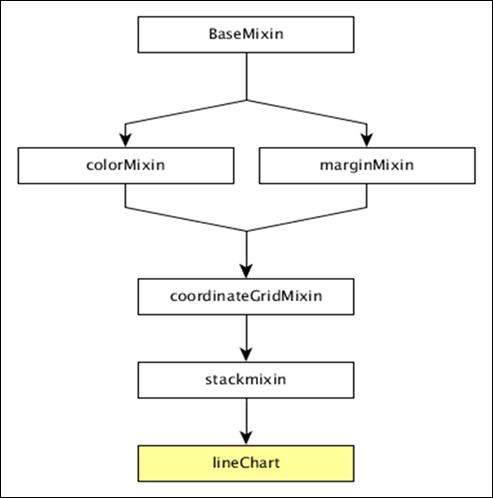# DC.js - Line Chart

#### Complete Python Prime Pack for 2023

9 Courses     2 eBooks

#### Artificial Intelligence & Machine Learning Prime Pack

6 Courses     1 eBooks

#### Java Prime Pack 2023

8 Courses     2 eBooks

A line chart is used to display information as a series of data points connected by straight lines. A data point represents two values, one plotted along the horizontal axis and another along the vertical axis. For example, the popularity of food items can be drawn as a line chart in such a way that the food item is represented along the x-axis and its popularity is represented along the y-axis. This chapter explains about line charts in detail.

## Line Chart Methods

Before moving on to draw a line chart, we should understand the dc.lineChart class and its methods. The dc.lineChart uses mixins to get the basic functionality of drawing a chart. The mixins used by dc.lineChart are as follows −

• dc.stackMixin
• dc.coordinateGridMixin

The complete class diagram of the dc.lineChart is as follows −The dc.lineChart gets all the methods of the above-specified mixins as well as it has its own methods to draw the line chart. They are explained as follows.

### dashStyle( [style])

This method is used to set the dash style for a line chart.

This method is used to get or set the radius (in PX) for dots displayed on the data points. It is defined as follows −

```chart.dotRadius = function (radius) {
if (!arguments.length) {
}
};
```

### interpolate( [i])

This method is used to get or set the interpolator for a line.

### renderArea( [area])

This method is used to get or set the render area.

### renderDataPoints( [options])

This method is used to render individual dots for each data point.

### tension( [tension])

This method is used get or set the tension for the lines drawn. It is in the range from 0 to 1.

### xyTipsOn( [xyTipsOn])

This method is used to change the mouse behavior of an individual data point.

## Draw a Line Chart

Let us draw a line chart in DC. To do this, we need to follow the steps given below −

### Step 1: Define a variable

Let us define a variable as shown below −

```var chart = dc.lineChart('#line');
```

Here, the dc.linechart function is mapped with the container having an id line.

### Step 2: Read the data

Read data from the people.csv file −

```d3.csv("data/people.csv", function(errors, people) {
var mycrossfilter = crossfilter(people);
}
```

Here, if we used the same dataset people.csv, the sample data file will be as follows −

```id,name,gender,DOB,MaritalStatus,CreditCardType
1,Damaris,Female,1973-02-18,false,visa-electron
2,Barbe,Female,1969-04-10,true,americanexpress
3,Belia,Female,1960-04-16,false,maestro
4,Leoline,Female,1995-01-19,true,bankcard
5,Valentine,Female,1992-04-16,false,
6,Rosanne,Female,1985-01-05,true,bankcard
7,Shalna,Female,1956-11-01,false,jcb
8,Mordy,Male,1990-03-27,true,china-unionpay

...............
................
................
```

### Step 3: Create an age dimension

Now, create dimension for age as shown below −

```var ageDimension = mycrossfilter.dimension(function(data) {
return ~~((Date.now() - new Date(data.DOB)) / (31557600000))
});
```

Here, we assigned the age from the Crossfilter data.

The ~~ is a double NOT bitwise operator. It is used as a faster substitute for the Math.floor() function.

Now, group it using the reduceCount() function, which is defined below −

```var ageGroup = ageDimension.group().reduceCount();
```

### Step 4: Generate a chart

Now, generate a line chart using the coding given below −

```chart
.width(800)
.height(300)
.x(d3.scale.linear().domain([15,70]))
.brushOn(false)
.yAxisLabel("Count")
.xAxisLabel("Age")
.dimension(ageDimension)
.group(ageGroup)
.on('renderlet', function(chart) {
chart.selectAll('rect').on('click', function(d) {
console.log('click!', d);
});
});

chart.render();
```

Here,

• Chart width is 800 and height is 300.

• The d3.scale.linear function is used to construct a new linear scale with the specified domain range [15, 70].

• Next, we set the brushOn value to false.

• We assign the y-axis label as count and x-axis label as age.

• Finally, group the age using ageGroup.

### Step 5: Working example

The complete code listing is shown in the following code block. Create a web page line.html and add the following changes to it.

```<html>
<title>DC.js Line Chart Sample</title>
<link rel = "stylesheet" type = "text/css" href = "css/bootstrap.min.css">
<link rel = "stylesheet" type = "text/css" href = "css/dc.css"/>

<script src = "js/d3.js"></script>
<script src = "js/crossfilter.js"></script>
<script src = "js/dc.js"></script>

<body>
<div>
<div id = "line"></div>
</div>

<script language = "javascript">
var chart = dc.lineChart('#line');

d3.csv("data/people.csv", function(errors, people) {
var mycrossfilter = crossfilter(people);

var ageDimension = mycrossfilter.dimension(function(data) {
return ~~((Date.now() - new Date(data.DOB)) / (31557600000))
});
var ageGroup = ageDimension.group().reduceCount();

chart
.width(800)
.height(300)
.x(d3.scale.linear().domain([15,70]))
.brushOn(false)
.yAxisLabel("Count")
.xAxisLabel("Age")
.dimension(ageDimension)
.group(ageGroup)
.on('renderlet', function(chart) {
chart.selectAll('rect').on('click', function(d) {
console.log('click!', d);
});
});
chart.render();
});
</script>
</body>
</html>
```

Now, request the browser and we will see the following response.